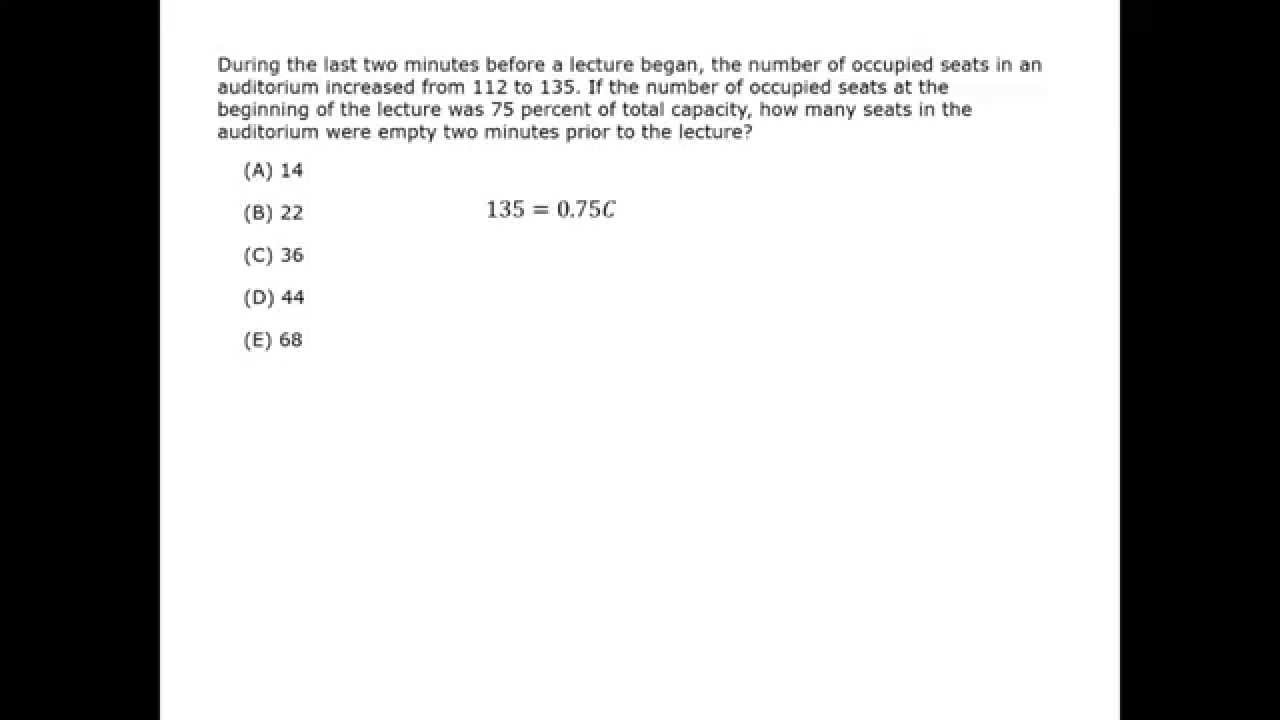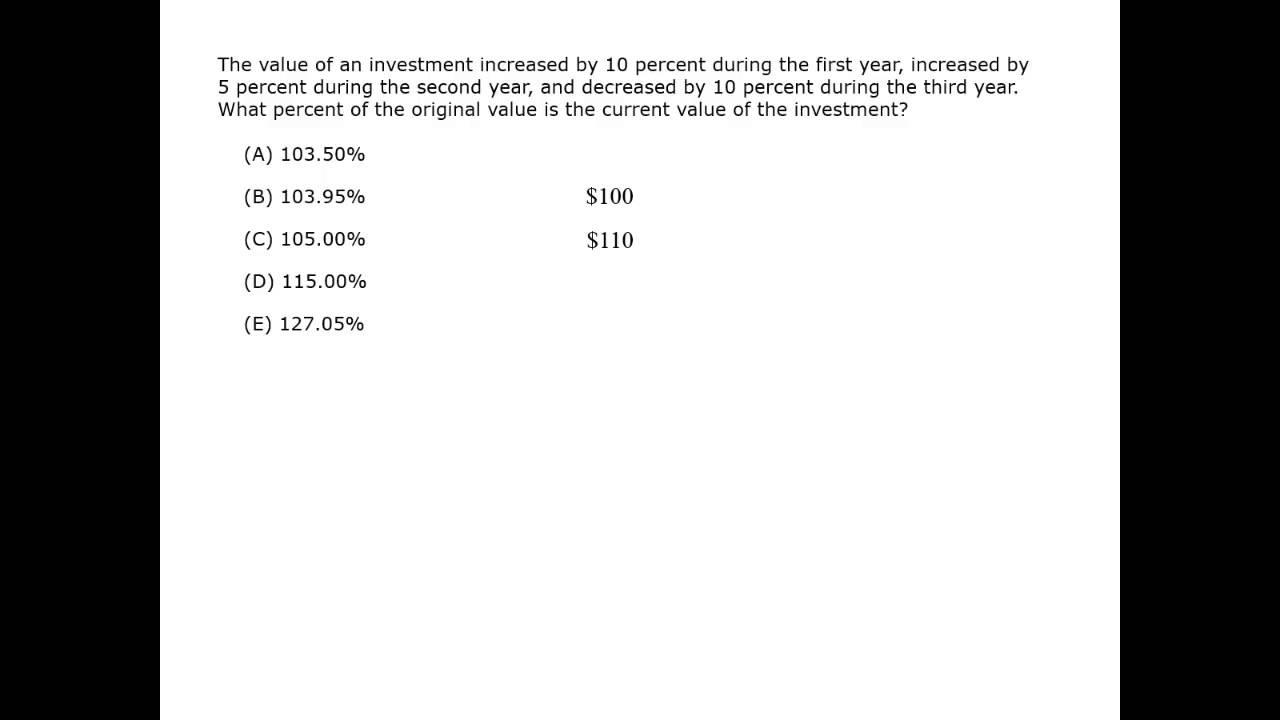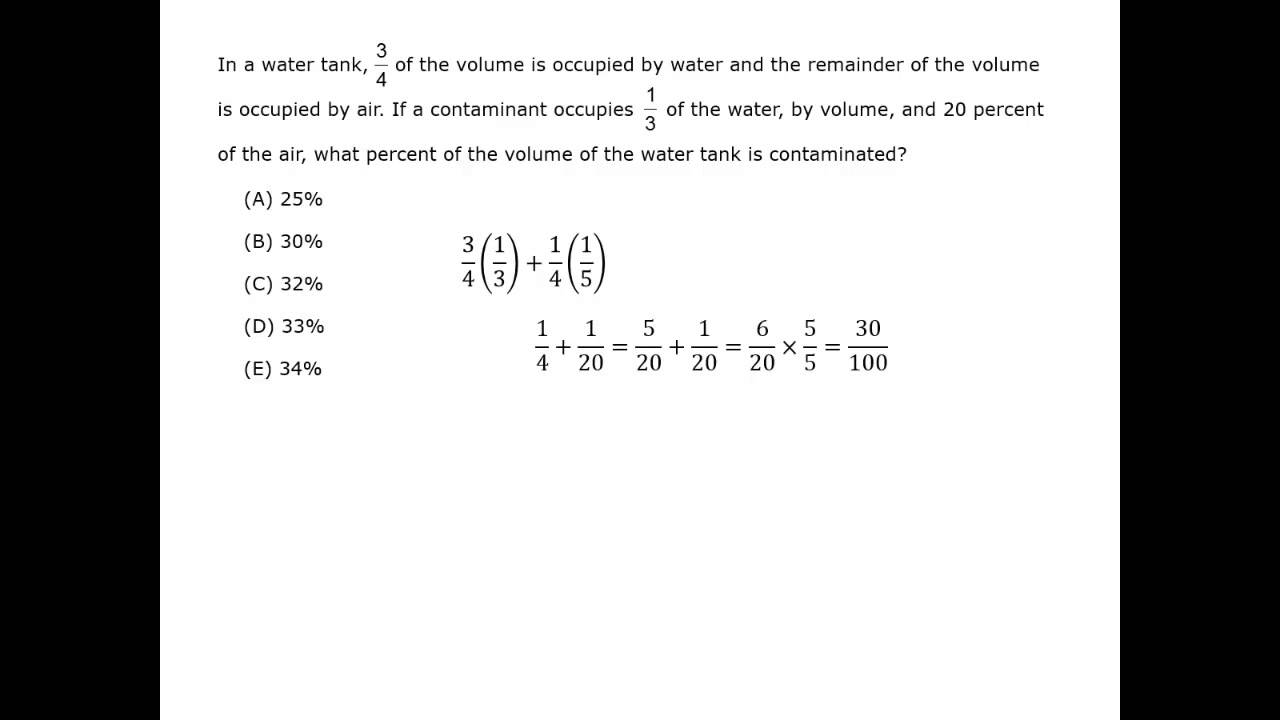#### Percent & Conversions

The word percent means per 100. It is a fraction whose denominator is 100. For example, 26% is equivalent to the fraction \dfrac{26}{100}.

A percentage is used to compare values. It can describe the relationship of a part to a whole, or it can describe a magnitude of change.

Decimal to Percent
To change a decimal number to a percentage, multiply by 100; this moves the decimal point 2 places to the right. Then add the percent symbol.

0.32 = 32%
0.07 = 7%
1.75 = 175%

Percent to Decimal
To change a percentage to a decimal, divide by 100; this moves the decimal point 2 places to the left. Then remove the percent symbol.

25% = 0.25
90% = 0.90 = 0.9
32.1% = 0.321
100% = 1

Fraction to Decimal
You can convert a fraction to a decimal by dividing the numerator by the denominator. Sometimes a quicker method is to change the fraction so it has 100 as the denominator.

{21}{100} = 0.21

\dfrac{43}{50} = \dfrac{86}{100} = 0.86

\dfrac{13}{16} = \dfrac{13}{16} = 0.8125

Decimal to Fraction
To convert decimal to a fraction, move the decimal point 2 places to the right and use 100 as the denominator. Then simplify the fraction. If there is still a decimal in the numerator, move the decimal point to the right as needed, and add a 0 to the denominator for each place value.

0.55 = \dfrac{55}{100} = \dfrac{11}{20}

2.18 = \dfrac{218}{100} = \dfrac{109}{50} = 2 \,\dfrac{9}{50}  or  2.18 = 2

\dfrac{18}{100} = 2\dfrac{9}{50}

0.123 = \dfrac{12.3}{100} = \dfrac{123}{1000}

Note: There is a chart at the end of this section with common fraction and decimal conversion values.

Fraction and Percent
To change a percentage to a fraction, change the percentage to a decimal, then the decimal to a fraction.

34% = 0.34 = \dfrac{34}{100} = \dfrac{17}{50}

Correspondingly, to change a fraction to a percentage, change the fraction to a decimal, then the decimal to percent.

\dfrac{18}{90} = \dfrac{2}{10} = 0.2 = 20%

Convert 4% into a decimal and a fraction in lowest terms.

### Solution

To convert 4% into a decimal, move the decimal point two places to the left. This requires adding a leading 0.
4% = 0.04

To express 4% as a fraction, put it over a denominator of 100, and then simplify the fraction.
\dfrac{4}{100} = \dfrac{4}{4} × 25 = \dfrac{1}{25}

#### Multiplying by Percent

Multiplying by a percentage is a way to find a “part” of the original. Multiplying by a percentage is the same as multiplying by the decimal equivalent.

15% of 40 = 15% × 40 = 0.15 × 40 = 6
8% of 25 = 8% × 25 = 0.08 × 25 = 2

Quick trick: Multiplying by 10%

To get 10% of anything, simply slide the decimal point one place to the left.
3456 × 10% = 345.6

For 20%, slide the decimal point one place to the left, then multiply by 2.
3456 × 20% = 345.6 × 2 = 691.2

The same pattern holds true for 30%, 40%, 60%, 70%, 80% and 90%. But for 50%, simply divide by 2.

What is 30% of 210?

### Solution

You are trying to find the “part” of 210 that is equal to 30%.

30% of 210 = 210 × 30%  Change to multiplication.
= 21 × 3  Slide the decimal point one place to the left, then multiply by 3.
= 63

30% of 210 is 63.

You may also be given two numbers (a part and a whole) and asked to find the percentage of the whole represented by the part. One way to do this is to use a proportion, which sets 2 fractions equal to each other. Change the given numbers to a fraction and set it equal to a fraction with denominator 100. Solve for the numerator and that will be the percentage.

What percent of 24 is 9?

### Solution

You are trying to find the percent that matches the given fraction.

\dfrac{part}{whole} = \dfrac{percent}{100} so \dfrac{9}{24} =\dfrac{\textit{x}}{100}

Method 1

900 = 24x Use cross multiplication.

x = \dfrac{900}{24} = 3 × 3 × 4 × \dfrac{25}{2} × 3 × 4 = 3 × \dfrac{25}{2} = \dfrac{75}{2} = 37.5

Method 2

Start by simplifying the fraction.
\dfrac{9}{24} = \dfrac{3}{8}

Since you know \dfrac{1}{8} = 0.125, you can quickly calculate \dfrac{3}{8} = 3(0.125) = 0.375 = 37.5%

Percent Multipliers

#### Percent

Best viewed in landscape mode

8 questions with video explanations

100 seconds per question#### Percent Increase and Decrease

Percentages can be used to describe the magnitude of change. To find the percentage of change, you compare the new amount to the original amount.

Percent increase: If the price of a $30 item increases by 10%, the new price is the original$30 plus 10% of the $30 original,$33. This is 110% or 1.1 times the original price. “Increase” may go by other names such as the “markup” from “wholesale” (cost from the factory) to “retail” (cost to the public).

### Solution

Notice that the price is being marked up by 120%, not to 120%.

The amount of the markup is 120% of $110 so it’s 1.2 × 110 =$132.
The selling price is then the original price plus the markup, so $110 +$132 = $242. Another way to calculate the selling price is 120% + 100% = 220%, so 2.2 ×$110 = $242 A college bookstore purchases trade books on a 30% margin, i.e., it purchases a trade book for 30% less than its retail price. What is the percentage markup from the wholesale price? ### Solution The wholesale price is the retail price minus 30% of the retail price. wholesale = retail – (30% of retail) = (100% – 30%) × retail = 70% × retail So the wholesale price is 70% of the retail price. But don’t stop there. It is a common GMAT trick to require you to use the result of one part of a question to get the final answer. This question is asking for the markup from wholesale to retail. To make calculations easier, use$100 rather than a variable as the retail price of a trade book. Then the wholesale is 70% × retail = 70% × $100 =$70.

new – \dfrac{original}{original} = \dfrac{percent}{100}

retail – \dfrac{wholesale}{wholesale} = \dfrac{markup}{100}

100 – \dfrac{70}{70} = \dfrac{markup}{100}
\\[2ex]\dfrac{30}{70} = \dfrac{3}{7} = \dfrac{42.8}{100} = \dfrac{markup}{100}

So the markup from wholesale to retail is 43%.

Find the number of residents in a city if 20% of them, or 6200 people, ride bicycles.

### Solution

Let R be the number of residents. Translate the words into an equation.

20% of R is 6200, so 0.2R = 6200

Change the decimal to a fraction.

0.2R = 6200

(\dfrac{2}{10})= 6200

To divide by a fraction, multiply by the reciprocal.

R = 6200 × (\dfrac{10}{2}) = 62,000 = 31,000

The city has 31,000 residents.

#### Sale Prices

In determining sale prices, be careful not to mix up the amount taken off the original price with the new, sale price.

Kent pays 20% tax on income between $10,000 and$20,000 and 30% on income over $20,000. The first$10,000 is tax-free. If he paid $14,000 in taxes, what was his income? ### Solution Let Kent’s income be k. Divide his income into the tax brackets: • For$0 to $10,000, there is no tax. • The$10,000 between $10,000 to$20,000 is taxed at 20%.
• Income beyond $20,000 is taxed at 30%. So, the equation is: 10,000 (0%) + 10,000 (20%) + (k – 20,000)(30%) = 14,000 0 + 2000 + 0.3(k – 20,000) = 14,000 2000 + 0.3k – 6000 = 14,000 0.3k = 18,000 k = 18,000 × (\dfrac{10}{3}) = 60,000 Kent’s income was$60,000.

#### Percent

Best viewed in landscape mode

9 questions with video explanations

100 seconds per question#### Mixture Problems

How many gallons of pure water must be added to 100 gallons of a 4% saline solution to produce a 1% saline solution?

### Solution

Let x be the gallons of pure water to be added.
In 100 gallons of a 4% saline solution, there are:

0.04(100) = 4 gallons of salt.

In the 1% solution, the total number of gallons will be 100 + x. The amount of salt will remain constant at 4 gallons.

0.01(100 + x) = 4
1 + 0.01x = 4
0.01x = 3
x = \dfrac{3}{0.01} = 300

300 gallons of water need to be added to produce a 1% saline solution.

#### Taking a Percentage of a Percentage

What happens if you take a percentage of a number and then take a percentage of that new value? You are just multiplying the new total by a second percentage. This is a common GMAT trick.

If the price of a stock starts at $100, increases by 10% during the first year, then increases again by another 20% in the second year, what is the stock’s final price? ### Solution In the first year,$100 × 110% = $110. For the second year,$110 × 120% = $132. The stock price would be$132.

Notice that this is not the same price change as 10% + 20% = 30%.
A 30% increase would have been resulted in a $130 price. #### Fractions and Percentile Joe’s portfolio lost 80% of its value, then gained back 10% of its value. What was Joe’s final percentage loss? ### Solution Use$100 for the original value to help solve the question.

The portfolio lost 80%.
100% − 80% = 20%, so $20 was left. That$20 gained back 10% of its value, so $20 × 110% =$22.

So 22% is the percentage of the original investment that he still has.

Subtract 100% − 22%. The final percentage loss is 78%.

Notice that this is not 100% − 80% + 10% = -70%, or a 70% loss.

A backpack was marked for sale at 30% off, and an additional 20% was taken off at the register. If the original price was $50, what was the final purchase price? ### Solution The discount is not 30% + 20% = 50%. 30% off means you pay 70%. Another 20% off means paying 80% of the 70%.$50 × 0.8 × 0.7 = $50 × 0.56 =$28

A way to check this is to calculate the dollar amount coming off the price.
30% off of $50 = 0.3 ×$50 = $15. So the purchase price before getting to the register is$50 × $15 =$35.
20% off of $35 = 0.2 ×$35 = $7 So the final purchase price is$35 × $7 =$28.

#### Common Conversions

1 = 100%
3⁄4 = 75%
1⁄2 = 50%
1⁄4 = 25%

1⁄10 = 10%
3⁄10 = 30%
7⁄10 = 70%
9⁄10 = 90%

1⁄5 = 2⁄10 = 20%
2⁄5 = 4⁄10 = 40%
3⁄5 = 6⁄10 = 60%
4⁄5 = 8⁄10 = 80%

1⁄3 = 33.33
2⁄3 = 66.66

For many other denominators, you can remember the first value then multiply to get other values.
For example, if you remember that \dfrac{1}{8} = 0.125, then \dfrac{3}{8} = 3 × 0.125 = .375

\dfrac{1}{6} = 16.66%

\dfrac{5}{6} = 83.66%

\dfrac{1}{7} = 14.28%

\dfrac{2}{7} = 28.56%

\dfrac{3}{7} = 42.85%

\dfrac{4}{7} = 57.14%

\dfrac{5}{7} = 71.42%

\dfrac{6}{7} = 85.71%

\dfrac{1}{8} = 0.125 = 12.5%

\dfrac{3}{8} = 0.375 = 37.5%

\dfrac{5}{8} = 0.625 = 62.5%

\dfrac{7}{8} = 0.875 = 87.5%

\dfrac{1}{9} = 11.1%

\dfrac{2}{9} = 22.2%

\dfrac{4}{9} = 44.4%

\dfrac{5}{9} = 55.6%

\dfrac{7}{9} = 77.7%

\dfrac{8}{9} = 88.8%

\dfrac{1}{11} = 9.09%

\dfrac{2}{11} = 18.18%

\dfrac{3}{11} = 27.27%

\dfrac{4}{11} = 36.36%

\dfrac{5}{11} = 45.45%

\dfrac{6}{11} = 54.54%

\dfrac{7}{11} = 36.36%

\dfrac{8}{11} = 72.72%

\dfrac{9}{12} = 81.81%

\dfrac{10}{11} = 90.90%

\dfrac{1}{12} = 8.3%

\dfrac{5}{12} = 41.7%

\dfrac{7}{12} = 58.3%

\dfrac{11}{12} = 91.7%

#### Percent

Best viewed in landscape mode

8 questions with video explanations

100 seconds per question